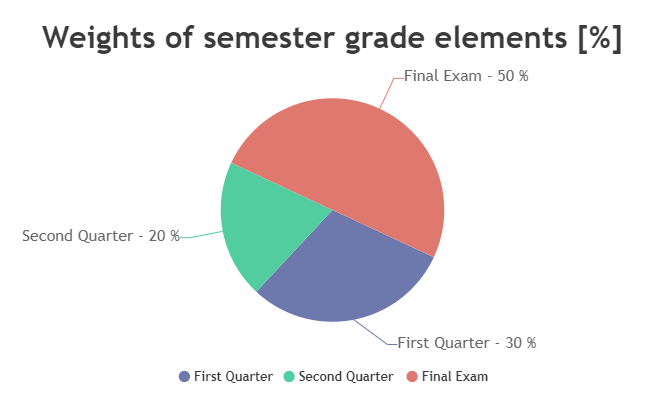Uh Oh! It seems you’re using an Ad blocker!

We always struggled to serve you with the best online calculations, thus, there's a humble request to either disable the AD blocker or go with premium plans to use the AD-Free version for calculators.

OrEnter the grades and the weighted semester grades in the tool and it will calculate the semester grades.

📕 First quarter

%

Percent of Semester Grade (weight) :

%

📗 Second quarter

%

Percent of Semester Grade (weight) :

%

🖋️ Final exam

%

Percent of Semester Grade (weight) :

%

Table of Content

Get the Widget!

Add this calculator to your site and lets users to perform easy calculations.

Feedback

How easy was it to use our calculator? Did you face any problem, tell us!

The final semester grade calculator calculates the overall semester grade, which helps you simplify the grading process. The grade semester calculator offers convenience and efficiency to both students and instructors. It gives 100% accurate calculations in less than a minute.

## How to Calculate Semester Grades?

You can calculate semester grades with the assessment of the student’s overall performance in the semester. The grade semester average calculator evaluates the percentage of marks for the first quarter, second quarter and final exam.

The percentage of each semester has a specific weightage in the final examination.

You can calculate semester grades as follows:

• Determine Weightings
• Calculate Assessment Scores
• Sum the Weighted Scores

## Formula for Weighted Semester Grade:

Our calculator uses the following formula to calculate weighted semester grades with accuracy:

Each of the grading systems can be different from other grading system. The semester grade calculator high school evaluates marks on the basis of the weight of the grade in each semester.

## Example:

Let’s suppose there is a first quarter worth 30% (0.30) with a score of 85 and a second quarter worth 20% (0.20) with an average score of 92. The final exam worth is 50% (0.50) with a score of 78. Then how to calculate your semester grade?

Sol:

Weighted Semester Grade = (0.30 * 85) + (0.20 * 92) + (0.50 * 78)**

Weighted Semester Grade = 25.5 + 18.4 + 39**

Weighted Semester Grade = 82.9 %

So, the weighted semester grade is 82.9 %.

The semester grading pie chart is represented asThe semester grade calculator is a valuable online tool for students and educators to calculate final semester grades precisely. The main reason beyond that there can be different grading systems and weightage of marks.

The grading systems vary somewhat depending on the institution. The semester grading chart used in schools and universities is as follows:

 Letter Grade Grade Points Percentage A+ 4.0 97-100 A 4.0 93-96 A- 3.7 90-92 B+ 3.3 87-89 B 3.0 83-86 B- 2.7 80-82 C+ 2.3 77-79 C 2.0 73-76 C- 1.7 70-72 D+ 1.3 67-69 D 1.0 63-66 D- 0.7 60-62 F 0.0 Below 60

There can be a difference between the weightage of each quarter. The 2 quarter exam calculator allows the user to adjust the weightage of each semester.

## How Does Semester Grade Calculator Work?

The semester exam calculator takes into account each quarter marks the final exam marks separately.

Let’s see how!

Input:

• Enter the grades of the first quarter, second quarter and final exam
• Enter the relative weightage of grades

Output:

• Pie Chart of Weightage

## FAQs:

### What is the Main Difference Between Cumulative GPA and Semester GPA?

The cumulative GPA is a calculation of all the courses in school or a college. The semester GPA is the average course grade for the current semester.

### How to Calculate the Final Grade in a Points-Based System?

• Determine the point values
• Record all the points earned
• Add points and possible points
• Divide the total points and possible points

Calculate semester grades on the basis of the points and then convert them into the percentage with the semester test calculator.

### What Is a 3.0 GPA?

A 3.0 unweighted GPA means a student has earned a B average across the course studied during a specific class.

### What Is the Highest GPA In the US?

A 4.0 GPA is equivalent to acquiring an A in all of your classes. This is the highest possible score.